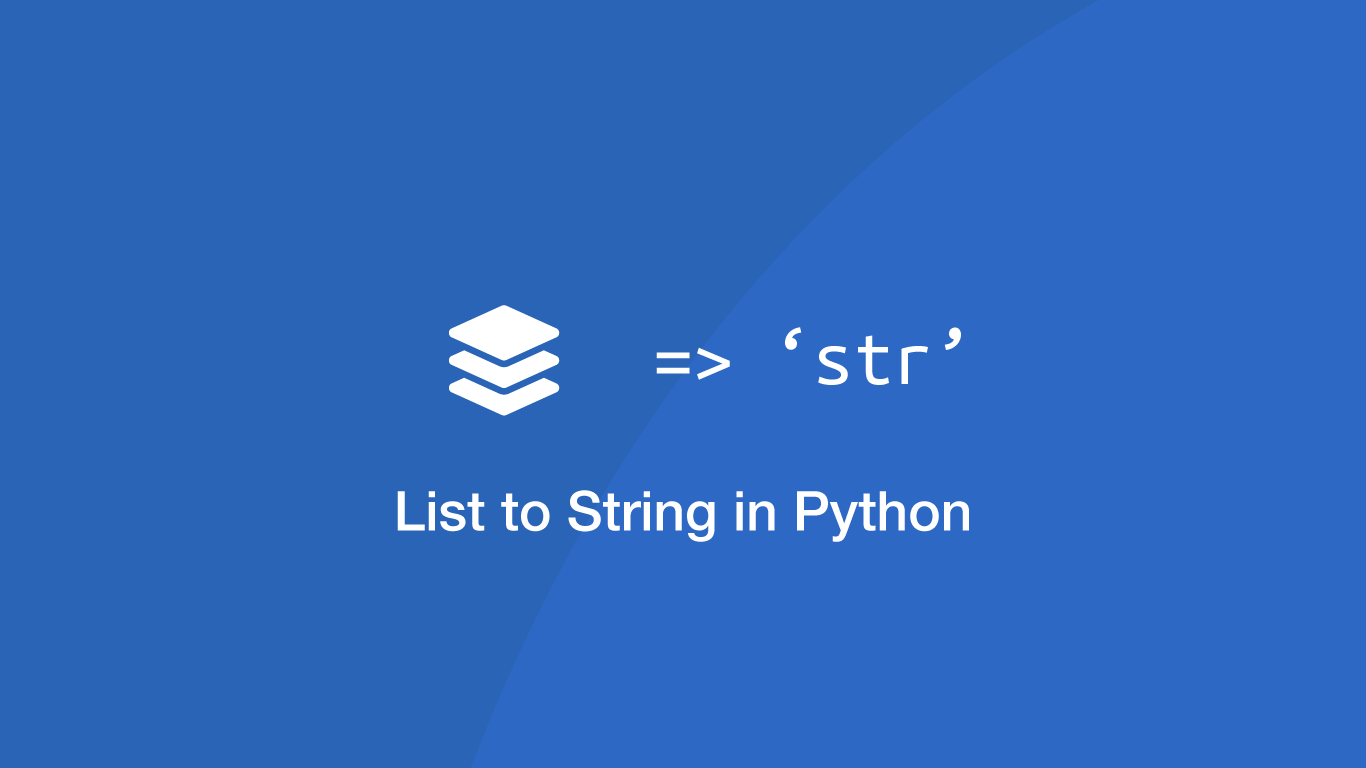# How to Convert a List to a String in PythonThere are multiple ways to approach converting a `list` to a string in Python, which we will explore in this tutorial. The method you choose might depend on the type of data inside the `list` or how the output string should be formatted.

While the examples below convert lists, the same principles can be applied to any other iterable data types such as tuples.

## The .join() Method

The easiest way to convert a `list` to a string is by using the `join()` method. To use it pass in the glue characters followed by a `.` (dot) and then the `join()` method with the `list` as the first argument.

``````fruits = ['apple', 'strawberry', 'orange', 'apricot']

glue = ' '

string = glue.join(fruits)

print(string)
``````
``````apple strawberry orange apricot
``````

## Converting a List of Numbers to a String

To convert a `list` of numbers to a comma-separated string, convert the numbers to strings using `str()` then strip the `[]` (square brackets) using `strip()`.

``````nums = [1,2,3]

string = str(nums).strip('[]')

print(string)
``````
``````1, 2, 3
``````

## Convert a List to a String Using List Comprehension

List comprehension is a shorthand way to loop through a `list` and do something with each element. In the example below, we are looping through a `list` containing numbers and strings, converting them all to strings in a `for` loop before passing the result to the `join()` method.

``````mixed = [1,2,3,'four','five']

string = ' '.join([str(e) for e in mixed])

print(string)
``````
``````1 2 3 four five
``````

## Convert a List of Numbers and Strings Using map()

This example, like the previous one, can convert a `list` containing mixed data into a string. It works by using the `map()` method to convert each element in the list to a string before passing the result to the `join()` method.

``````mixed = [1,2,3,'four','five']

string = ' '.join(map(str, mixed))

print(string)
``````
``````1 2 3 four five
``````

## Conclusion

You now know several ways to convert lists into strings and glue them together with different characters using the `join()` method. As mentioned at the start of the post, you can use these methods to convert other iterable data types such as tuples and objects.

#### Related Tutorials### Convert a List to Lowercase in Python

September 21, 2021### How to Compare Strings in Python

September 20, 2020### How to Read a File Line by Line in Python

September 21, 2020### How to Parse JSON Data in Python (Read and Write)

September 18, 2020### How to use Sets in Python

September 15, 2020### How to Create Multi-Line Strings in Python

September 18, 2020